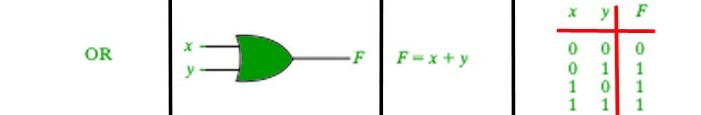GeeksforGeeks App
Open AppBrowser
Continue

# || operator in Java

|| is a type of Logical Operator and is read as “OR OR” or “Logical OR“. This operator is used to perform “logical OR” operation, i.e. the function similar to OR gate in digital electronics.One thing to keep in mind is the second condition is not evaluated if the first one is true, i.e. it has a short-circuiting effect. Used extensively to test for several conditions for making a decision.
Syntax:

```Condition1 || Condition2

// returns true if one of the conditions is true.
```

Below is an example to demonstrate || operator:
Example:

## Java

 `// Java program to illustrate``// logical OR operator` `import` `java.util.*;` `public` `class` `operators {``    ``public` `static` `void` `main(String[] args)``    ``{` `        ``char` `ch = ``'a'``;` `        ``// check if character is alphabet or digit``        ``// using || operator``        ``if` `(ch >= ``65` `&& ch <= ``90``            ``|| ch >= ``97` `&& ch <= ``122``)``            ``System.out.println(``                ``ch``                ``+ ``" is an alphabet."``);``        ``else` `if` `(ch >= ``48` `&& ch <= ``57``)``            ``System.out.println(``                ``ch``                ``+ ``" is a digit."``);``        ``else``            ``System.out.println(``                ``ch``                ``+ ``" is a special character."``);``    ``}``}`

Output:

```a is an alphabet.

```

My Personal Notes arrow_drop_up